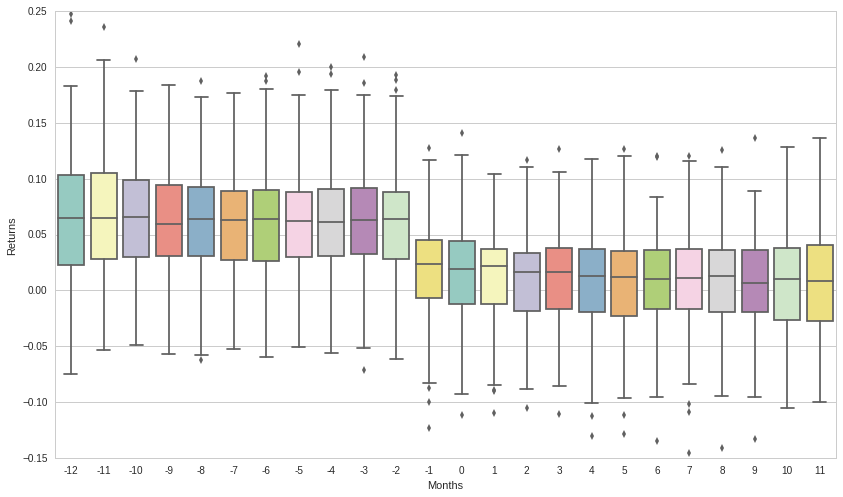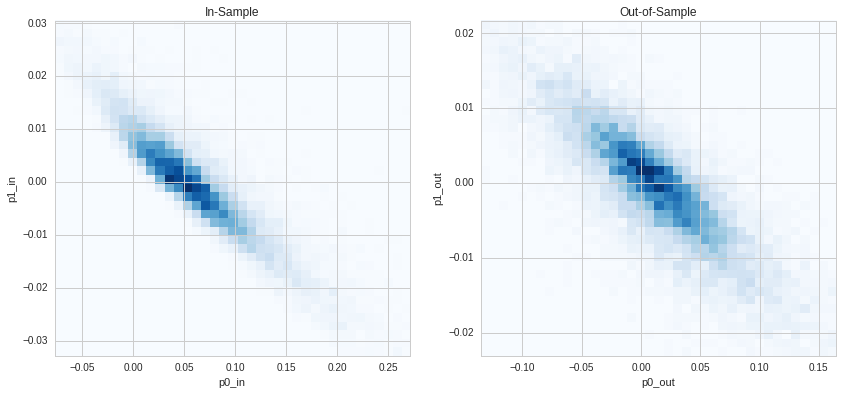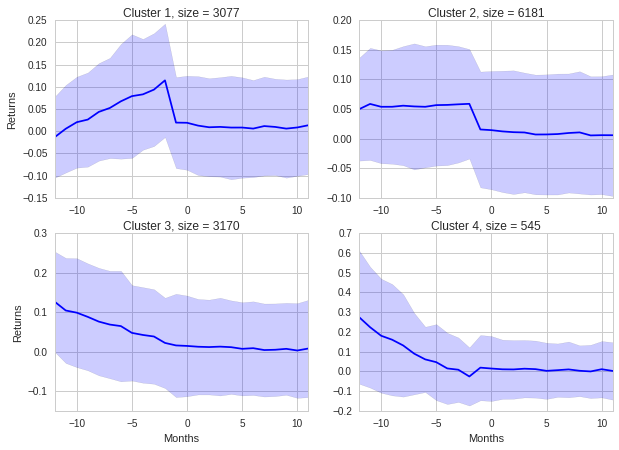Notebook

This notebook features the next Quantopian-based paper “Uncovering Momentum" that extends the previous "Momentum with Volatility Timing" study with a top-bottom analysis of the momentum strategy including a sequence of four steps: dissecting the momentum performance along bull/bear states and winners/losers deciles; identifying the unscaled momentum decile as a basic common block across conventional, time-series and dual momentum strategies; rolling the combined in/out-of-sample analysis, and clustering momentum decile time series. Combining the in- and out-of-sample analysis highlights the difference in performance levels between the two intervals and prompts a more asymmetrical version of the momentum definition like "achievement award for lucky winners". The small price of this award does not represent a challenge for the Efficient Market Hypothesis and defines a criterion for assessing the underlying momentum theories and models.

This notebook is related to Section IV focusing on time series clustering of the in/out-of-sample dataset and is structured as follows:

In :
import datetime
from time import time
import numpy as np
import pandas as pd
from pandas.tseries.offsets import BDay, BMonthBegin
import matplotlib.pyplot as plt
from matplotlib import cm
import seaborn as sns
from quantopian.pipeline import Pipeline
from quantopian.pipeline.data.builtin import USEquityPricing
from quantopian.pipeline.factors import CustomFactor, Returns, Latest, MarketCap
from quantopian.pipeline.classifiers import Classifier
import quantopian.pipeline.factors as Factors
from quantopian.research import run_pipeline
import alphalens as al
import pyfolio as pf
import empyrical as ep
from scipy.stats.mstats import winsorize
from statsmodels import regression
import statsmodels.api as sm
from sklearn.cluster import KMeans


## 1. Quantopian and Alphalens Processing¶

Following the previous post, this section is based on the Quantopian and Alphalens framework that is comprehensively described in Lecture 39: Factor Analysis with Alphalens. In comparisson with the lecture, however, the paper applies the Quantopian framework to the conventional winners-minus-losers momentum strategy that was documented by Jegadeesh and Titman (1993).

Defining time interval

In :
load_start_date, load_end_date = '2004-07-01', '2019-09-30'


Running pipeline with the Momentum class

In :
universe = QTradableStocksUS()

# The momentum factor is computed by ranking stocks according to
# their prior behavior over the course of 11 months with a 1 month lag

wl = 252
class Momentum(CustomFactor):
""" Conventional Momentum factor """
inputs = [USEquityPricing.close]
window_length = wl

def compute(self, today, assets, out, prices):
out[:] = (prices[-21] - prices[-wl])/prices[-wl]

def make_pipeline():
pipe = Pipeline()
pipe.set_screen(universe)
return pipe

pipe = make_pipeline()

results.dropna(inplace=True)

mom_scores = results['momentum']


Pipeline Execution Time: 39.01 Seconds

Calculating forward returns and quantile ranks with Alphalens

In :
# Retrieving prices for pipeline assets
asset_list = results.index.levels

mom_data = al.utils.get_clean_factor_and_forward_returns(factor=mom_scores,
prices=prices,
quantiles=10,
periods=(1, 21))

Dropped 0.7% entries from factor data: 0.7% in forward returns computation and 0.0% in binning phase (set max_loss=0 to see potentially suppressed Exceptions).
max_loss is 35.0%, not exceeded: OK!


## 2. Defining Bull and Bear Market States¶

Bull and bear market are identified similarly to Daniel and Moskowitz (2016) based on the cumulative two year past returns with negative values representing the latter market state.

In :
mkt_start_date = datetime.datetime.strptime(load_start_date, '%Y-%m-%d') - BDay(1)
mkt_prices = get_pricing('SPY', start_date=mkt_start_date, end_date=load_end_date, fields='price')
mkt_rtns = mkt_prices.pct_change(periods=1)[1:]

In :
def moving_cum_rtns(symbol, window=24*21):
return (1. + symbol).rolling(window).agg(lambda x: x.prod()) - 1.

mkt_cum_rtns = moving_cum_rtns(mkt_rtns)


## 3. Switching from Daily to Monthly Data¶

Selecting monthly dates from daily data with interval of 21 business days

In :
days = mom_data.index.levels.unique()
d21s = days.values[::21][:-1]

mom_d21 = mom_data.loc[list(d21s)]


## 4. Rolling In/Out-of-Sample Asset Time Series for the Bull Market¶

The paper explores the momentum effect with two in/out-of-sample approaches. The first (Section III) rolls a family of decile portfolios having the same stocks and ranking intervals but different one-month holding periods. The second approach (Section IV) works on the stock level. Both versions compile a composite dataset covering the 12-month in/out-of-sample intervals. The former period includes the 11-month ranking interval plus lagged one month in accordance with the momentum strategy.

This section shows the second approach that is implemented with the function rolling_inout_rnk. The procedure consists of an outer loop and two inner loops. The first inner loop selects an intersection of winners tradable stocks spanning the in/out-of-sample intervals. Then, the second inner loop creates the corresponding in/out-of-sample dataframe with all selected stocks for the specific formation date. The outer loop rolls both inner loops across the defined time horizon and concatenates dataframes.

In :
def rolling_inout_rnk(mom_input, d21s, window = 12, mom_rnk=10):

days = []; dfs = []

for t in range(window, d21s.size - window):

# select assests in the formation date t for the specified rank

xs_t = mom_input.xs(d21s[t])
assets = xs_t[xs_t['factor_quantile'] == mom_rnk].index

# select the subset/intersection of tradable assets
# in the [-window, window] interval

inter_assets = assets.values

for dt in range(-window, window):
xs_dt = mom_input.xs(d21s[t+dt]).loc[inter_assets]
xs_dt_nan = xs_dt[xs_dt['21D'].notnull()]
inter_assets = np.intersect1d(inter_assets, xs_dt_nan.index.values)

# in/out dataframe for each month

df_xs_dt = pd.DataFrame(xs_t.loc[inter_assets]['factor_quantile'])

for dt in range(-window, window):
xs_dt = mom_input.xs(d21s[t+dt]).loc[inter_assets]
df_xs_dt[str(dt)] = xs_dt['21D']

df_xs_dt.drop(['factor_quantile'], axis=1, inplace=True)

days.append(d21s[t])
dfs.append(df_xs_dt)

df_output = pd.concat(dfs, keys=days)
df_output.index.rename('date', level=0, inplace=True)

return df_output


Defining the window size for the in/out-of-sample intervals

In :
window = 12


Dropping the before/after 12-month window to avoid overlap with the bear market states

In :
bear_indices = np.argwhere(mkt_bear_mask_d21 == True)[:,0]
d21s_bull = np.delete(d21s, np.arange(bear_indices - window, bear_indices[-1] + window + 1))


Creating the in/out-of-sample dataframe

In :
mom10_rtns = rolling_inout_rnk(mom_d21, d21s, window, mom_rnk=10)
mom10_rtns_bull = mom10_rtns.loc[list(d21s_bull)]


## 5. In/Out-of-Sample Momentum Decile Analysis¶

This section reproduces the results generated with the first portfolio-level approach from Section III in the paper. Note: The notebook computes equally-weighted rather than value-weighted deciles as in the portfolio-level approach.

The figure below presents the corresponding boxplots for the top decile summarizing the monthly distributions of portfolio returns over the in/out-of-sample intervals. Tables B1 and B2 in Appendix B in the paper compliment the figure with quantitative results for winners and losers encompassing the annual mean, alpha and beta parameters. As shown in the boxplots, the difference in performance between the ranking and out-of-sample intervals prompts clarification of the conventional momentum definition such as "winners continue to win" with more accurate asymmetrical versions like "lucky winners accumulate an achievement award". The small price of this award does not represent a challenge for the Efficient Market Hypothesis and defines a criterion for assessing the underlying momentum theories and models.

In :
grouper = [mom10_rtns_bull.index.get_level_values('date')]
mom10_inout_means = mom10_rtns_bull.groupby(grouper).aggregate('mean')

In :
mom10_inout_boxplot = mom10_inout_means.unstack().reset_index()
mom10_inout_boxplot.columns = ['Months', 'Date', 'Returns']
ax = sns.boxplot(x='Months', y='Returns', data=mom10_inout_boxplot, palette='Set3', order=mom10_inout_means.columns)## 6. Clustering In/Out-of-Sample Stock Time Series¶

Moving down to the stock level connects the in- and out-of-sample analysis to studies on predictability of cross-section returns and various explanatory variables such as investor sentiment (Baker and Wurgler, 2006; Huang et al., 2015) and firm characteristics (Lewellen, 2015; Green et al., 2017).

The paper proposes to extend the scope of past studies by emphasizing the analysis of stock temporal behavior. For example, the stock-level time series of ranking and out-of-sample intervals can be further classified with parameters calculated from the linear regression: $r*{im}$ = $p0*{i}$ + $p1*{i}$ ∗ $m$ + $e*{im}$, where $r_{im}$ is stock return observed at month m.

In :
def calc_in_out_lr(x, x_in, x_out):

p0s_in = []; p1s_in = []
p0s_out = []; p1s_out = []

for i in range(0, len(x.index)):

asset_i = x.iloc[i]

y_in = asset_i.values[0:11]
y_out = asset_i.values[12:24]

lm_in = regression.linear_model.OLS(y_in, x_in).fit()
p0s_in.append(lm_in.params)
p1s_in.append(lm_in.params)

lm_out = regression.linear_model.OLS(y_out, x_out).fit()
p0s_out.append(lm_out.params)
p1s_out.append(lm_out.params)

df = pd.DataFrame(list(zip(p0s_in, p1s_in, p0s_out, p1s_out)), index=x.index,
columns=['p0_in', 'p1_in', 'p0_out', 'p1_out'])

return df

In :
x_in = sm.add_constant(np.arange(0, 11)) # x coordinates for ranking interval
x_out = sm.add_constant(np.arange(0, 12)) # x coordinates for out-of-sample interval

grouper = [mom10_rtns_bull.index.get_level_values('date')]
df_lr = mom10_rtns_bull.groupby(grouper).apply(lambda x: calc_in_out_lr(x, x_in, x_out))


The two-dimensional histograms based on the $p0$ and $p1$ parameters subsequently provide an additional perspective to the portfolio-based boxplots of Section 5.

In :
def plot_hist2d(ax, p0_name, p1_name, title):
x = winsorize(df_lr[p0_name].values, limits=[0.01, 0.01])
y = winsorize(df_lr[p1_name].values, limits=[0.01, 0.01])

x_bins = np.linspace(np.min(x),np.max(x), 40)
y_bins = np.linspace(np.min(y),np.max(y), 40)

ax.hist2d(df_lr[p0_name], df_lr[p1_name], bins=[x_bins, y_bins], cmap=cm.Blues)
ax.set_xlabel(p0_name)
ax.set_ylabel(p1_name)
ax.set_title(title)

fig, axs = plt.subplots(1, 2, sharey=False, figsize=(14, 6))
plot_hist2d(axs, 'p0_in', 'p1_in', 'In-Sample')
plot_hist2d(axs, 'p0_out', 'p1_out', 'Out-of-Sample')The plots for both the ranking and out-of-sample interval display similar shapes stretching across a negatively tilted line. The $p1$ coordinates for the shape centers equal approximately to zero and subsequently the $p0$ coordinates correspond to the centers of the mean distributions. Such distributions represent easy targets for sorting and clustering techniques. Consequently, the distribution of the ranking interval was further split into four clusters based on the k-means multivariate approach.

In :
n_clusters = 4

km_data_in = np.matrix([df_lr['p0_in'].values, df_lr['p1_in'].values])

km_in = KMeans(n_clusters=n_clusters, init='k-means++', max_iter=300)
km_in.fit(km_data_in.T)

y_km_in = km_in.predict(km_data_in.T)


Creating lookup table for reordering cluster labels

In :
idx = np.argsort(km_in.cluster_centers_.sum(axis=1))
lut = np.zeros_like(idx)
lut[idx] = np.arange(4)


Associating each stock with a corresponding cluster

In :
def set_cluster(x, cluster_labels):
global indx
df = pd.DataFrame(index=x.index)
df['cluster'] = cluster_labels[indx : indx + len(x.index)]
indx += len(x.index)
return df

indx = 0
grouper = [mom10_rtns_bull.index.get_level_values('date')]
df_clusters = mom10_rtns_bull.groupby(grouper).apply(lambda x: set_cluster(x, lut[y_km_in]))


Combining the in/out-of-sample stock time series with cluster labels

In :
df_da = pd.concat([mom10_rtns_bull, df_clusters], axis=1)


Calculating monthly return means and top/bottom sigmas for each cluster

In :
cl_centers = np.zeros(shape=(n_clusters, 2*window))
cl_top = np.zeros(shape=(n_clusters, 2*window))
cl_bottom = np.zeros(shape=(n_clusters, 2*window))
cl_counts = np.zeros(n_clusters)

for i in range(0, n_clusters):
df_cl = df_da[df_da.cluster == i]
cl_counts[i] = df_cl.shape
for j in range(-window, window):
cl_centers[i][window+j] = np.mean(df_cl[str(j)])
cl_top[i][window+j] = np.mean(df_cl[str(j)]) + np.std(df_cl[str(j)])
cl_bottom[i][window+j] = np.mean(df_cl[str(j)]) - np.std(df_cl[str(j)])


Plotting the corresponding cluster results

In :
def plot_cluster(ax, n_cluster):
ts = range(-window,window)
ax.plot(ts, cl_centers[n_cluster], '-', color='blue')
ax.fill_between(ts, cl_bottom[n_cluster], cl_top[n_cluster], facecolor='blue', alpha=0.2)
ax.set_title('Cluster {0}, size = {1}'.format(n_cluster+1, int(cl_counts[n_cluster])))
ax.margins(x=0)

fig, axs = plt.subplots(2, 2, figsize=(10, 7))

plot_cluster(axs[0,0], 0)
axs[0,0].set_ylabel('Returns')
plot_cluster(axs[0,1], 1)
plot_cluster(axs[1,0], 2)
axs[1,0].set_xlabel('Months')
axs[1,0].set_ylabel('Returns')
plot_cluster(axs[1,1], 3)
axs[1,1].set_xlabel('Months')
plt.show()The cluster plots confirm and elaborate the results from Section 5. In general, temporal patterns can be described by the sampling of the momentum strategy. At the same time, they complement the in-out difference metric with the fine-grain clustering technique for testing underlining momentum models. Furthermore, time series clustering enables to facilitate this analysis by reducing the parameter heterogeneity (Greene, 2012) of explanatory variables. Finally, the application of the conventional clustering approaches can be further enhanced with the deep learning framework.